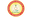Trending ▼   ResFinder# ICSE Prelims 2017 : Mathematics (Sri Siddhivinayaka Residential School (SSRS), Hattiangadi, Kundapur)

7 pages, 50 questions, 47 questions with responses, 73 total responses,00Usha Shetty Sri Siddhivinayaka Residential School (SSRS), Hattiangadi, Kundapur
+Fave Message
 Home > yoboy_utsav >   F Also featured on: School PageFormatting page ...

X hi I A N I)A R I) ' ' M A T 1114:M A T I :I T IM E 2H : 30Mln r IA Q u e s tio n So V tte m p t a ll th e q u , l e tio n s , I 1 th e g iv e n in e q u a tio n a n d r e r e s e n t th e s o lu tio p v e A RO LI NO M A R K S : 80 . in v e s ts 7 15 0 0 0 m an A fte r I) , one R a te y e a r h is o m o ne m o u n t fo r F ro m a pack 2 n d is d r a w A b la c k ii) A re d in te re s t p a y a b le a n n lta be f u m lly 4 ]6 , 8 0 0 F in d a m o u n ts to n R do m a t ra n n 13 1 ye a r f 52 p la y in g o Clack k in g s fe ll d o w I) y un th e n f in te r e s t ii) A c ard po an co m n se t o n c a rds , a e m a in in bla c k ja c k g p a c k is a re d qu een ll s h u ffle d F in d th e p r o b a b ility th a t th e iii) A c a rd n ace o r a N e ith e r iv ) c a rd d fa c e w e , a an d tw d th M an c a rd d ra w o a is n k in g qu e e n n or a k in g [4 ] Q a e tifm 2 to v e u u c rf va im a r x u s in l p la c e s g th e fo r m : (x u la a n 2 1) d g iv e y o u r 3x + 4 = a n s w e r c o r r e c t to tw o 0 [3 ] lu a te : irl ;4 L S in 5 6 2 la n 18 ta n 72 ' c *M 3I Scanned by CamScannerFormatting page ...

Top Contributors
to this ResPaperSaransh Srivastava(26)Sayantan karmakar(16)Mann Shah(15)Siddhi Nagwekar(11)Formatting page ...Formatting page ...Formatting page ...Formatting page ...Formatting page ...